# ISEE Middle Level Quantitative : How to find the area of a trapezoid

## Example Questions

### Example Question #221 : Quadrilaterals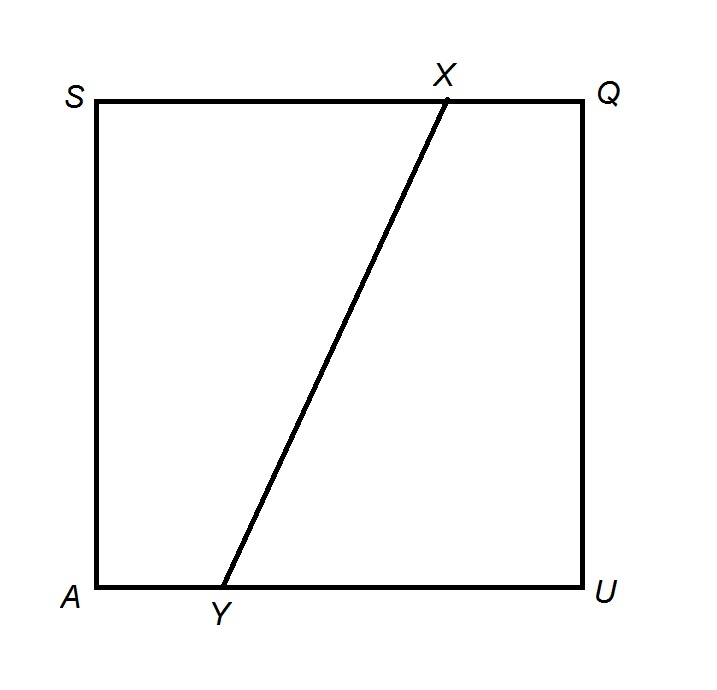Note: Figure NOT drawn to scale

The above figure shows Square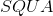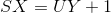Which is the greater quantity?

(a) The area of Trapezoid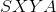(b) The area of Trapezoid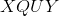(a) and (b) are equal

(a) is the greater quantity

(b) is the greater quantity

It is impossible to determine which is greater from the information given

(a) is the greater quantity

Explanation:

The easiest way to answer the question is to locate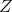on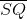such that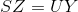: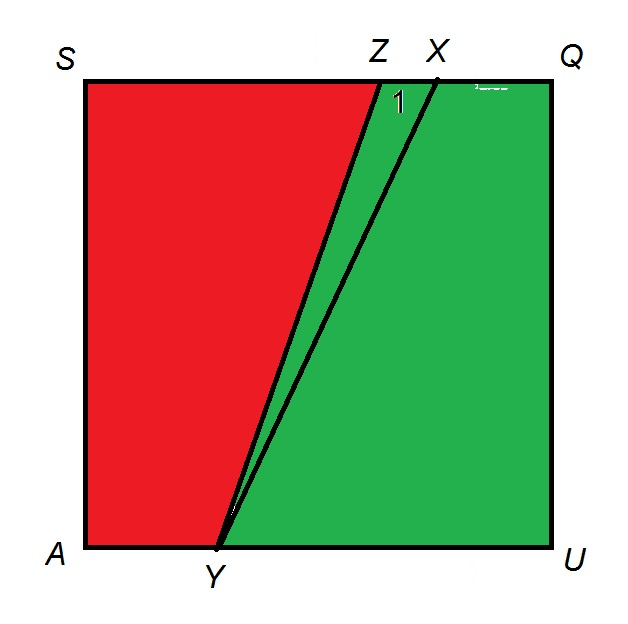Trapezoids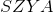and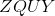have the same height, which is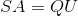. Their bases, by construction, have the same lengths -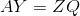and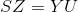. Therefore, Trapezoidsandhave the same area.

Since, it follows that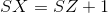, and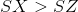. It follows that Trapezoidis greater in area than Trapezoidsand, and Trapezoidis less in area.